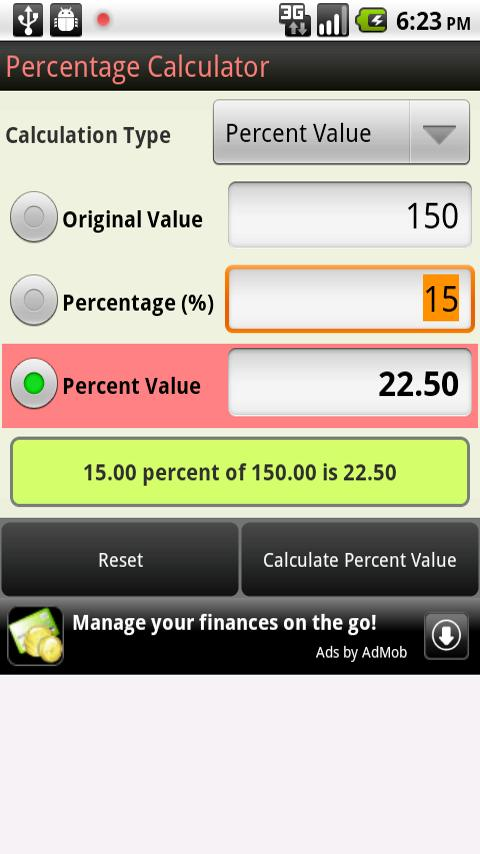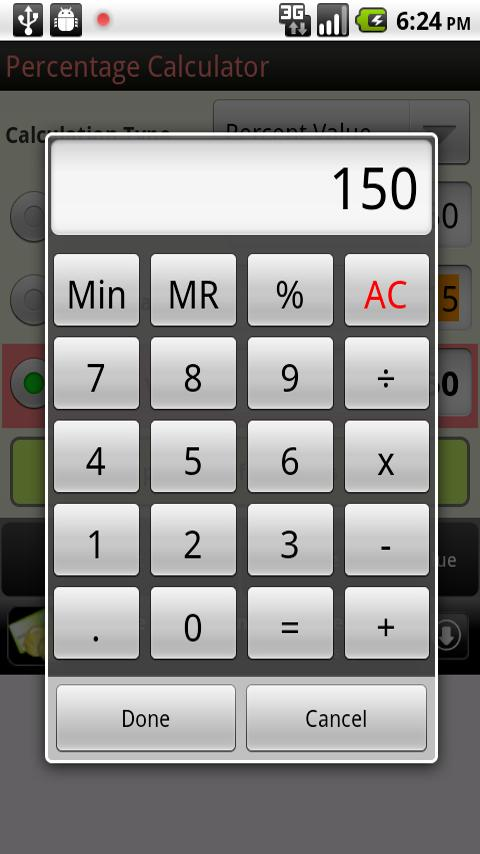# Percentage CalculatorThis is the ultimate percentage calculator app!

Use Percentage Calculator to quickly calculate:
1)Percent value & percentage
2)Decrease by X percent (discount or sale price)
3)Increase by X percent (markups or selling price)
4)Percentage change between two values

Percentage Calculator can also be used as a tip calculator.

### App Specification

• App Name: Percentage Calculator
• Rating: 5
• Apk: com.handyapps.percentcalc
• Cost: Free
• Developer: http://www.handy-apps.com
• Market: market://search?q=pname:com.handyapps.percentcalc